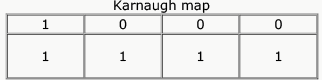Courses

# K Map And Logic Gates MCQ Quiz - 1

## 30 Questions MCQ Test RRB JE for Computer Science Engineering | K Map And Logic Gates MCQ Quiz - 1

Description
This mock test of K Map And Logic Gates MCQ Quiz - 1 for Computer Science Engineering (CSE) helps you for every Computer Science Engineering (CSE) entrance exam. This contains 30 Multiple Choice Questions for Computer Science Engineering (CSE) K Map And Logic Gates MCQ Quiz - 1 (mcq) to study with solutions a complete question bank. The solved questions answers in this K Map And Logic Gates MCQ Quiz - 1 quiz give you a good mix of easy questions and tough questions. Computer Science Engineering (CSE) students definitely take this K Map And Logic Gates MCQ Quiz - 1 exercise for a better result in the exam. You can find other K Map And Logic Gates MCQ Quiz - 1 extra questions, long questions & short questions for Computer Science Engineering (CSE) on EduRev as well by searching above.
QUESTION: 1

### Consider numbers represented in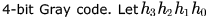be the Gray code  representation of a number n and let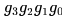be the Gray code of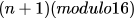value of the number .Which one of the following functions is correct?

Solution: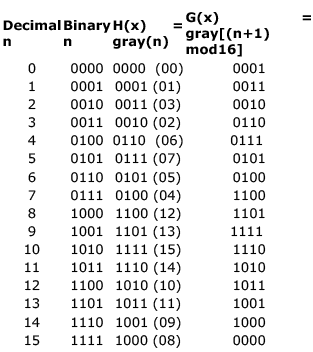We need to map min terms of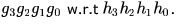Hence as highlighted g2 matches with option C
Edit : we have to map h(x) with g(x)...mod 16 is used in g(x) only because since we have 4 bits ,mamximum possible no that can be represented is 15..so after 15 we shouldn't get 16 and go back to 0.. thats why..
now mapping is simple...we just have to map such that h(x)---->g(x+1)
means if h represents gray code of 0 then g will represent gray code of 1
if h represents gray code of 1 then g will represent for 2 and so on....
for last number h(15) ,mod 16 actually comes into picture which will make it to represent as g(0)
so draw table as mentioned above... now write g as a function of f ..simply how we do minimisation...see the min terms..
be careful only in one thing here...
example... for 2..gray code representation is 0011 meaning 3 in decimal..so if we select this row as a min term(just an example) then we have selected 3 and not 2 ..means row numbers are not representing min terms...rest everything is fine!

QUESTION: 2

### For any natural number , an ordering of all binary strings of length n is a Gray code if it starts with 0n, and any successive strings in the ordering differ in exactly one bit (the first and last string must also differ by one bit). Thus, for n = 3, the ordering (000, 100, 101, 111, 110, 010, 011, 001) is a Gray code. Which of the following must be TRUE for all Gray codes over strings of length n?

Solution:

1). In the question it is stated that -> Thus, for n=3, the ordering (000, 100, 101, 111, 110, 010, 011, 001) is a Gray code.
2). We have to find orderings such that they start with 0n , must contain all bit strings of length n and successive strings must differ in one bit.
Option c&d are clearly wrong.
Now, consider n=1. The only Gray code possible is {0,1}. Hence no of Gray code = odd for n=1.
For n=2 only two Gray code exists {00, 10, 11, 01} and {00, 01, 11, 10}. Thus no of Gray code = even for n=2.
Thus it is not that gray codes could be only even or only odd.
Hence option e is correct.

*Answer can only contain numeric values
QUESTION: 3

### A half adder is implemented with XOR and AND gates. A full adder is implemented with  two half adders and one OR gate. The propagation delay of an XOR gate is twice that of an AND/OR gate. The propagation delay of an AND/OR gate is 1.2 microseconds. A 4-bit-ripple-carry binary adder is implemented by using four full adders. The total propagation time of this 4-bit binary adder in microseconds is ______.

Solution: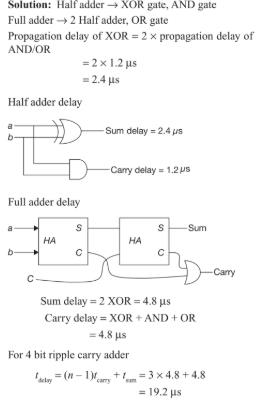QUESTION: 4

Consider the binary code that consists of only four valid codewords as given below: 00000, 01011, 10101, 11110
Let the minimum Hamming distance of the code p and the maximum number of erroneous bits that can be corrected by the code be q. Then the values of p and q are

Solution:

00000(code1),01011(code2),10101(code3),11110(code4)
Haming distance = min of all hamming distances.
which is 3 b/w (code1) and (code2) so p = 3
Now to correct d bit error we need hamming distance = 2d+1
so 2d+1 = 3 will gives d= 1.

QUESTION: 5

In the IEEE floating point representation the hexadecimal value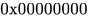corresponds to

Solution: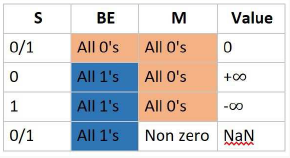QUESTION: 6

The following bit pattern represents a floating point number in IEEE 754 single precision format

1 10000011 101000000000000000000000

The value of the number in decimal form is

Solution:

Sign bit is 1 -> number is negative
Exponent bits- 10000011
Exponent is added with 127 bias in IEEE single precision format.
So, Actual exponent = 10000011 - 127 = 131 - 127 = 4
Mantissa bits- 101000000000000000000000
In IEEE format, an implied 1 is before mantissa, and hence the actual number is
-1.101 * 24
=- (11010)2 = - 26

QUESTION: 7

The decimal value 0.5 in IEEE single precision floating point representation has

Solution:

(B) is the answer. In IEEE uses normalized representation and hence an implicit '1' is used before the decimal point. So, if mantissa is 0000..0
it would be treated as 1.000..0
and hence the exponent need to be -1 for us to get 0.1 which is the binary representation of 0.5.
More into IEEE floating point representation:

QUESTION: 8

A Boolean function f is to be realized only by NOR gates. Its K-map is given below: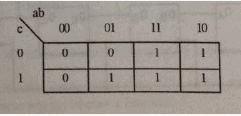The realization is

Solution:

A. can't be ans. ans wants without solving ??
Two Max Terms are: (a+b)(a+c) so "a" is common here only possibility is option D. ----------------------------------------------------------------------------------------------------------------------------------
-----------
Kmap will give min terms = a[4 1's circle] + bc[2 1's box] Option D ckt will give  = (a+b) (b+c) = a+bc

QUESTION: 9

What is the equivalent Boolean expression in product-of-sums form for the Karnaugh map given in Fig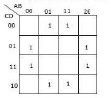Solution:

If all the empty cells are filled with '0' and then the POS expression is calculated as: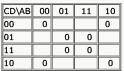Then the POS expression of f = (D+B)(D'+B') = BD' + B'D

QUESTION: 10

Which of the following expressions is in the product-of-sums form?

Solution:
QUESTION: 11

Which of the following functions implements the Karnaugh map shown below?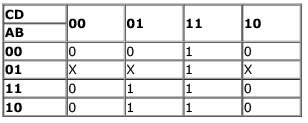Solution:

QUESTION: 12

The prime implicant which has at least one element that is not present in any other implicant is known as

Solution:

Essential prime implicants are prime implicants that cover an output of the function that no combination of other prime implicants is able to cover.

QUESTION: 13

Given the following karnaugh map, which one of the following represents the minimal Sum-Of-Products of the map?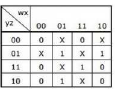Solution: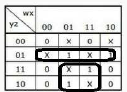QUESTION: 14

Minimum sum of product expression for f(w,x,y,z) shown in Karnaugh-map below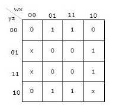Solution:

m4,m6,m12,m14 form one quad x'z So f=x'z+z'x

QUESTION: 15

The boolean function for a combinational circuit with four inputs is represented by the following Karnaugh map.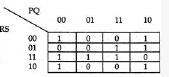Which of the product terms given below is an essential prime implicant of the function?

Solution:

essential prime implicants which r grouped only by only one method or way so in above question cornor's ones r grouped by only one method

QUESTION: 16

Consider the following expression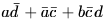Which of the following Karnaugh Maps correctly represents the expression?

Solution:
QUESTION: 17

Consider the following expression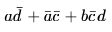Which of the following expressions does not correspond to the Karnaugh Map obtained for the given expression?

Solution:

ad'   [fill minterm in K-map in front for a and d' ]similarly fill all minterms  for ad'+a'c' +bc'd , resulting K-map will be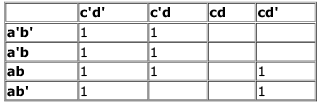option a) c'd'+ ad' + abc' + a'c'd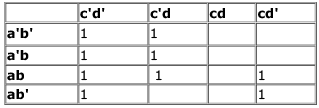s equivalent to given expression option b) a'c' + c'd' + ad' + abc'd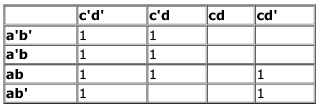is equivalent to given expression.
option c) a'c' + ad' + abc' + c'd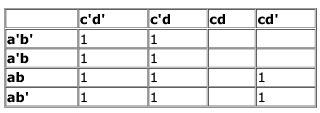is not equivalent to given expression.
option d) b'c'd' + acd' + a'c' + abc'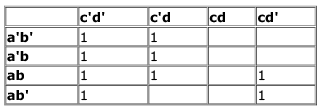is equivalent to given expression.

QUESTION: 18

In the Karnaugh map shown below, X denotes a don’t care term. What is the minimal form of the function represented by the Karnaugh map?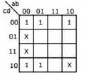Solution: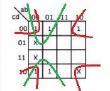Value for First one is a'd' and value for 2nd one is b'd'.

QUESTION: 19

What is the minimal form of the Karnaugh map shown below? Assume that X denotes a don’t care term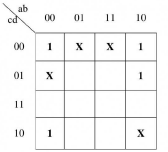Solution: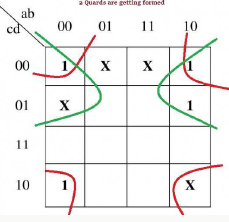Value for First one is b'd' and value for 2nd one is b'c'.So,Ans is option B

*Answer can only contain numeric values
QUESTION: 20

Consider the Karnaugh map given below, where X represents "don't care" and blank represents 0.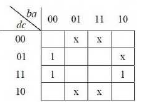Assume for all inputs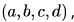the respective complements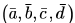are also available. The above logic is implemented using 2-input NOR gates only. The minimum number of gates required is ____________ .

Solution:

From K-map simplification we get the min-term as CA' . So We can simplyfy it for NOR gate expression
i.e. C' NOR A = (C'+A)' = CA'
Now complemented inputs are also given to us so for 2 input NOR gate we need only 1 NOR gate.

*Multiple options can be correct
QUESTION: 21

Choose the correct alternatives (more than one may be correct) and write the corresponding letters only:

All digital circuits can be realized using only

Solution:

NOR gate, NAND gate, Multiplexers and Half adders can also be used to realise all digital circuits.

QUESTION: 22

Which one of the following circuits is NOT equivalent to a 2-input XNOR (exclusive NOR) gate?

Solution: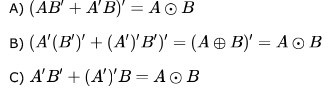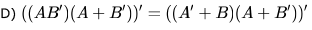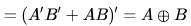So, Ans D)

QUESTION: 23

The Boolean function obtained by adding an inverter to each and every input of an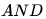gate is:

Solution: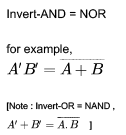QUESTION: 24

The capacity of a memory unit is defined by the number of words multiplied by the number of bits/word. How many separate address and data lines are needed for a memory of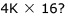?

Solution:

ROM memory size =2m x n
m=no. of address lines  n= no. of data lines
given  4K x 16
= 22 x 210 x 16
= 212 x 16 address lines =12
data lines= 16

QUESTION: 25

A circuit outputs a digit in the form of 4 bits. 0 is represented by 0000, 1 by 0001, …, 9 by 1001. A combinational circuit is to be designed which takes these 4 bits as input and outputs 1 if the digit > 5, and 0 otherwise. If only AND, OR and NOT gates may be used, what is the minimum number of gates required?

Solution:

Answer should be (B) As according to question .. truth table will be like
A B C D    f
0 0 0 0      0
0 0 0 1     0
0 0 1 0     0
0 0 1 1     0
0 1 0 0    0
0 1 0 1    1
0 1 1 0    1
0 1 1 1    1
1 0 0 0     1
1 0 0 1     1
1 0 1 0     dont care
1 0 1 1    dont care
1 1 0 0     dont care
1 1 0 1    dont care
1 1 1 0    dont care
1 1 1 1    dont care
using this truth table we get  3 sub cube which are combined with following minterms  A (8,9,10,11,12,13,14,15) , BD( 5,13,7,15) and BC(6,7,14,15)
SO f = A+ BD +BC= A+ B(C+D)
SO minimum gate required 2 OR gate and 1 AND gate = 3 minimum gate ...

QUESTION: 26

What is the minimum number of NAND gates required to implement a 2-input EXCLUSIVE-OR function without using any other logic gate?

Solution:
QUESTION: 27

What is the minimum number of gates required to implement the Boolean function (AB+C) if we have to use only 2-input NOR gates?

Solution:

given boolean function is
f = AB + C
= (A+C) . (B +C)
=((A+C)' +(B+C)')'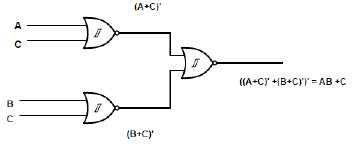therefore 3 NOR gate required .

QUESTION: 28

Consider the following Boolean function of four variables: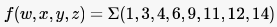The function is

Solution:

The K-map would be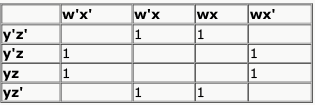So, the minimized expression would be x'z + xz'.
So, option B.

QUESTION: 29

Consider the following Boolean function of four variables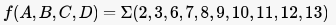The function is

Solution: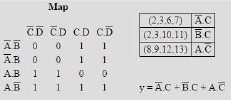QUESTION: 30

The simplified SOP (Sum of Product) from the Boolean expression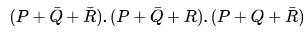is

Solution: